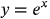1
37
views
61e
Problem

Problem 61e

Chapter 1: Functions and ModelsTextbook ExpertVerified Tutor
18 Oct 2021

Given information

The given equation is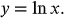Step-by-step explanation

Step 1.

Draw the graph of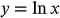and its reflected graph about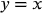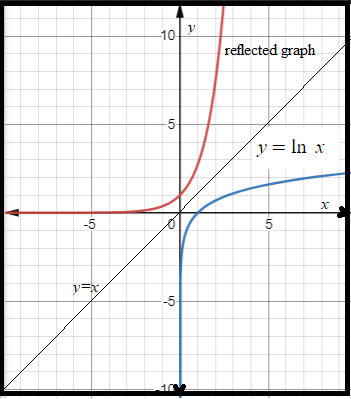The equation of the reflected graph is